# Algebra II : Functions as Graphs

## Example Questions

1 3 Next →

### Example Question #21 : Functions As Graphs

True or false: The graph of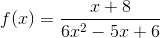has as a horizontal asymptote the graph of the equation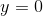.

False

True

True

Explanation:is a rational function in simplest form whose denominator is a polynomial with degree greater than that in its numerator (2 and 1, respectively). The graph of such a function has as its horizontal asymptote the line of the equation.

### Example Question #21 : Functions As Graphs

True or false: The graph of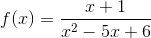has as a horizontal asymptote the graph of the equation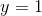.

False

True

False

Explanation:is a rational function in simplest form whose denominator has a polynomial with degree greater than that of the polynomial in its numerator (2 and 1, respectively). The graph of such a function has as its horizontal asymptote the line of the equation.

### Example Question #23 : Functions As Graphs

True or false: The graph of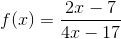has as a horizontal asymptote the graph of the equation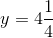.

True

False

False

Explanation:is a rational function whose numerator and denominator are polynomials of the same degree (1). As such, it has as a horizontal asymptote the line of the equation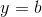, whereis the quotient of the coefficients of the highest-degree terms of its numerator and denominator. Consequently, the horizontal asymptote ofis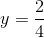or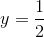.

### Example Question #22 : Functions As Graphs

True or false: The graph of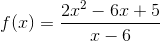has as a horizontal asymptote the line of the equation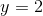.

True

False

False

Explanation:is a rational function whose numerator is a polynomial with degree greater than that of the polynomial in its denominator (2 and 1, respectively). The graph of such a function does not have a horizontal asymptote.

### Example Question #25 : Functions As Graphs

Which of the following equations is that of an oblique asymptote of the graph of the function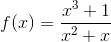?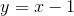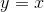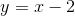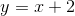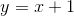Explanation:

To find an oblique asymptote of a rational function whose numerator has higher degree than its denominator, as is the case here, divide the former by the latter, as follows: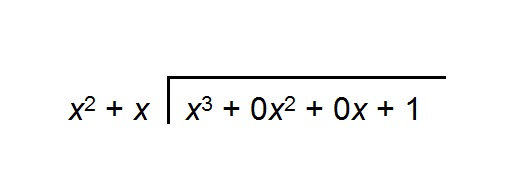Note that "missing" terms have been inserted in the dividend as terms with zero coefficients.

Divide the leading term of the dividend by that of the divisor: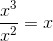Place this in the quotient, and multiply this by the divisor: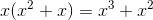Subtract this from the dividend. The figure should look like this: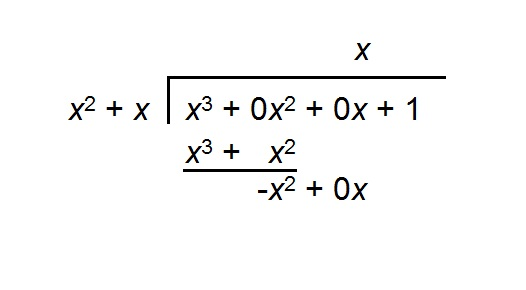Repeat with the difference: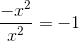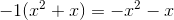The figure now looks like this: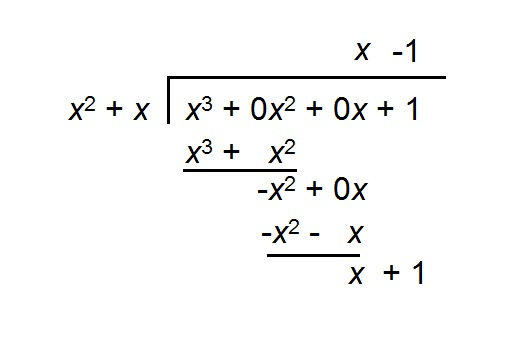The difference has degree less than that of the divisor, so the division is finished. The equation of the oblique asymptote of the graph ofis taken from the quotient, and is the line of the equation.

### Example Question #26 : Functions As Graphs

Which is a vertical asymptote of the graph of the function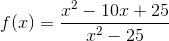?

(a)(b)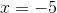Both (a) and (b)

Neither (a) nor (b)

Cannot be determined

(b) only

(a) only

(b) only

Explanation:

The vertical asymptote(s) of the graph of a rational function such ascan be found by evaluating the zeroes of the denominator after the rational expression is reduced

First, factor the numerator and denominator.

The numerator is a perfect square trinomial and can be factored as such: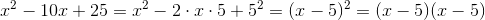The denominator can be factored as the difference of squares: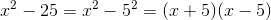Rewriteas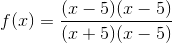The expression can be reduced by cancellingin both halves: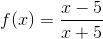Set the denominator equal to 0 and solve: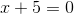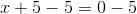The only asymptote is therefore the line of the equation### Example Question #27 : Functions As Graphs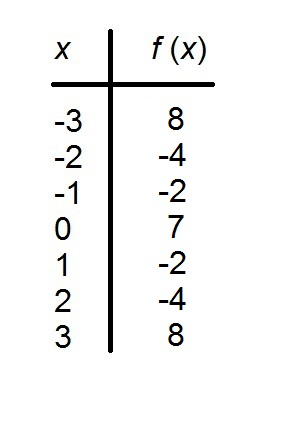The above table refers to a functionwith domain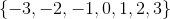.

Is this function even, odd, or neither?

Cannot be determined

Even

Neither

Odd

Even

Explanation:

A functionis odd if and only if, for everyin its domain,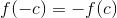; it is even if and only if, for everyin its domain,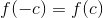. We can see that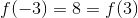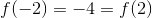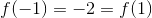Of course,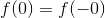.

Therefore,is even by definition.

### Example Question #21 : Functions And Graphs

Which of the following equations is that of an oblique asymptote of the graph of the function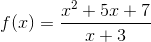?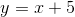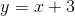The graph ofdoes not have an oblique asymptote.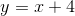Explanation:

To find an oblique asymptote of a rational function whose numerator has higher degree than its denominator, as is the case here, divide the former by the latter, as follows: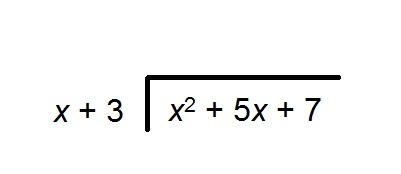Divide the leading term of the dividend by that of the divisor: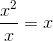Place this in the quotient, and multiply this by the divisor: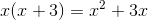Subtract this from the dividend. The figure should look like this: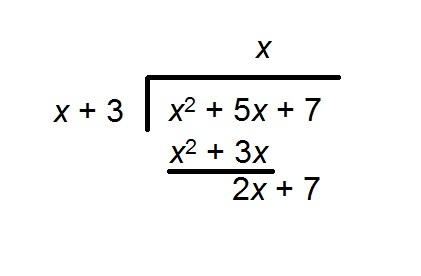Repeat with the difference: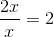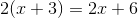The figure now looks like this: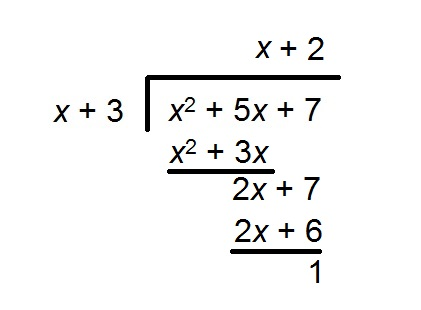The difference has degree less than that of the divisor, so the division is finished. The oblique asymptote is the quotient,1 3 Next →

### All Algebra II Resources